# -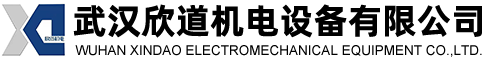# 027-81808005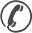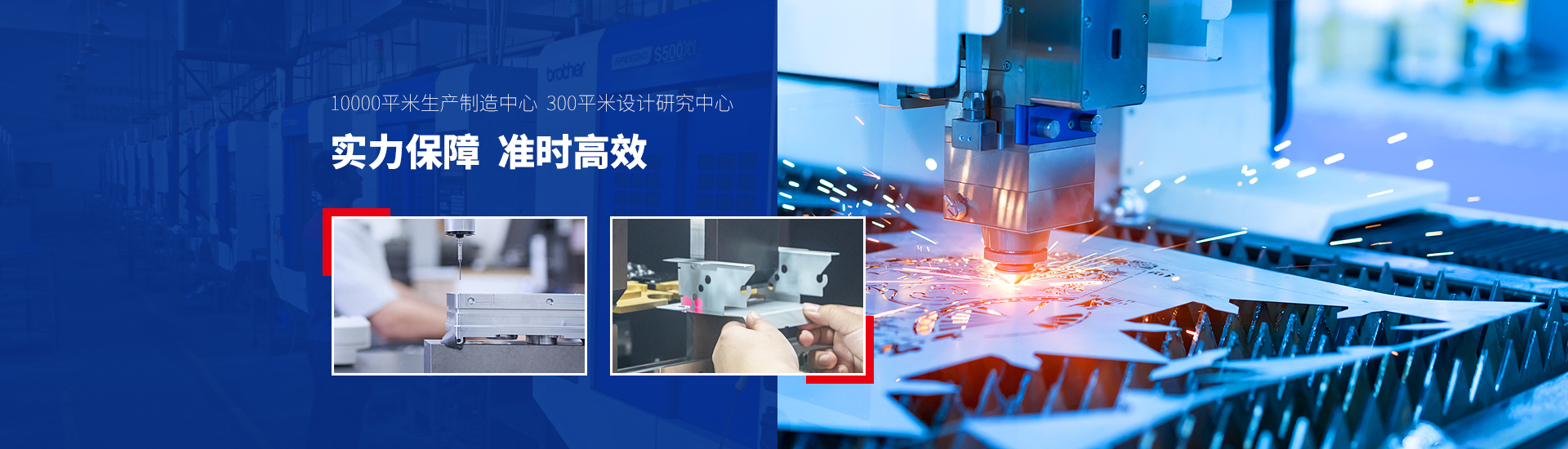13971605056

QQ：381730685

## 一、准备工时(R)

1、准备工时：指作业员熟悉图纸、开机、换程式、换刀模具、备料、首检、移料等时间的总和。各工序具体的定额如下表：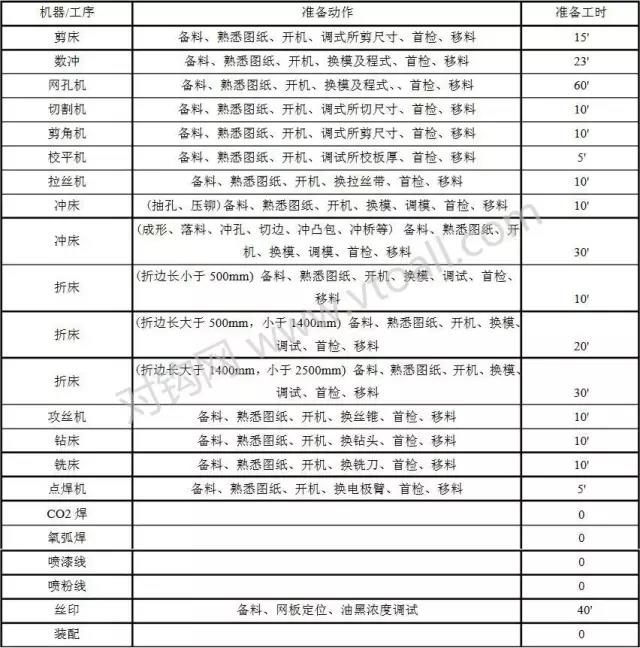2、在工时定额时，可根据实际情况对以上的准备工时作以适当的调整。

## 二、单件工时(V)

1、单件工时(V)：一位熟练工作者以规定的作业方法和工具完成一件合格料品所需的时间。

2、上下料时间、机器/人加工效率及辅助时间定额汇总表：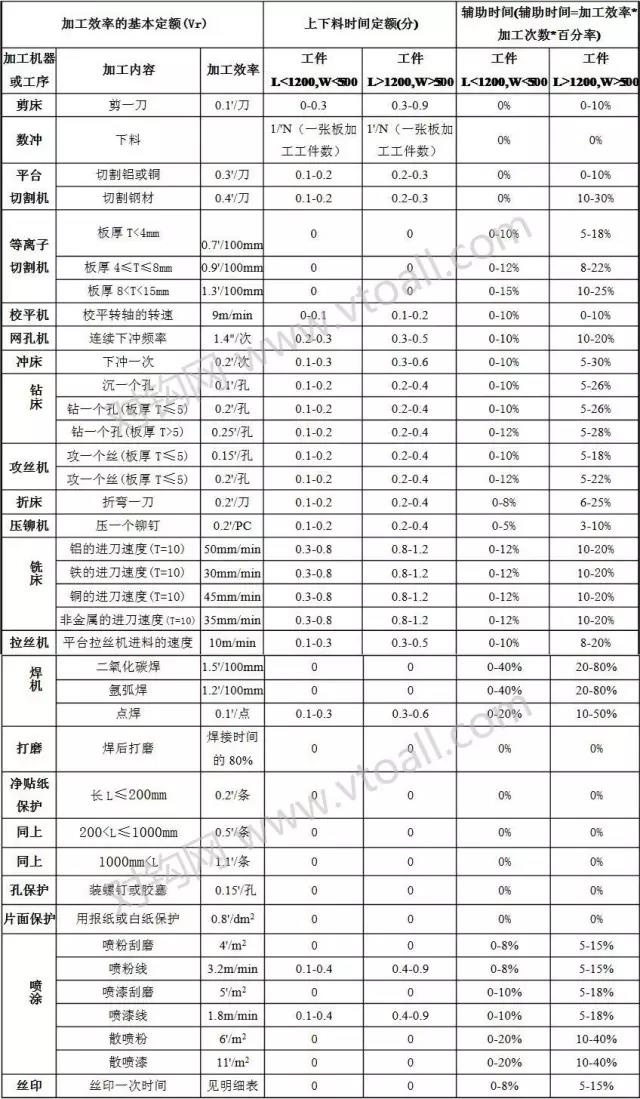3、宽放率标准定额：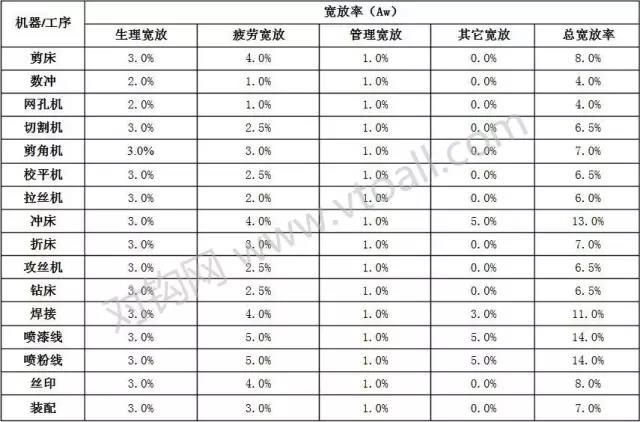4、各工序/机器单件工时具体定额

（1）剪床

V=Ts+(N*V)*(1+Aw)

N=剪料刀数

（2）数冲

a. V = Ts+[(C+D)/*V+E*H+F*K]*(1+Aw)

• C=工件外周长

• D=工件内孔周长(孔径大于60mm)

• E=换刀次数

• F=孔数(孔径等于或小于60mm)

• V=切边速度0.15′/1000mm

• H=自动换刀速度0.15'/次

• K=冲孔速度(松散孔0.01'/下冲一次，密集孔0.01'/下冲4次，依模具而定)

b. 覆膜与去毛刺时间 = 数冲单件工时(V)*0.4，当数冲时间超过3'时：

• 当3'≤T≤5'时，覆/去时间为0.8'～1.2'

• 当5'≤T≤10'时，覆/去时间为1.2'～1.8'

• 当10'≤T≤20'时，覆/去时间为1.8'～2.5'

• 当20'≤T≤30'时，覆/去时间为2.5'～3.5'

c. 当加工工件板材厚度大于2.0时，其单件工时须乘以1.2的系数。

（3）切割机

a. 平台切割机：V=Ts+Vr*N*(1+Aw）+F(可根据材料截面的大小对其机器工效率作以适当的调整)

• N=下切刀数

b. 等离子切割机：V=Vr*L*(1+Aw)+F

• L=切割缝长

（4）网孔机

V=Ts+Vr*N*(1+Aw)+F

• N=下冲次料=孔总数/一次冲孔数

（5）冲床

a. 抽孔、压铆：V=Ts+Vr*N*(1+Aw)+F

• N=压铆数量或抽孔数量

• 当N>15时，其单件工时(V)须乘以0.8系数；

b. 成形、落料、冲孔、切边、冲凸包、冲桥等：V=Ts+Vr*N*(1+Aw)+F

• N=下冲次数

• 剪条料后落料的单件时间须乘以0.6系数；

c. 当料件长大于1200mm，宽大于500mm时，其机床加工效率应乘以2。

（6）钻床

a. 钻孔：V=Ts+Vr*N*(1+Aw)+F

• N=孔数当孔径D>6时，其加工效率(Vr)需乘以2。

b. 沉孔：V=Ts+Vr*N*(1+Aw)+F

• N=孔数

c. 当料件长大于1200mm，宽大于500mm时，其机床加工效率应乘以2。

（7）攻丝机

V=Ts+Vr*N*(1+Aw)+F

• N=孔数

• 当料件长大于1200mm，宽大于500mm时，其机床加工效率应乘以2。

（8）拉丝机

V=Ts+Vr*L*(1+Aw)+F（单面拉丝）

V=Ts+Vr*L*(1+Aw)+F（双面拉丝）

• L=工件长度

• 当料件长小于200mm，宽大于50mm时，其机床加工效率应乘以0.8系数。

• 当料件长大于1200mm，宽大于500mm时，其机床加工效率应乘以2。

（9）折床

V=Ts+Vr*N*(1+Aw)+F

• N=折弯刀数

• 当料件长小于200mm，宽大于100mm时，其机床加工效率应乘以0.6系数。

• 当料件长大于1200mm，宽大于500mm时，其机床加工效率应乘以2。

（10）铣床

V=Ts+Vr*L*(1+Aw)+F

• L=走刀路线长度

（11）二氧化碳焊/氩弧焊

V=Ts+Vr*L*(1+Aw)+F

• L=焊缝长度

（12）点焊

V=Ts+Vr*N*(1+Aw)+F

• N=焊点数量

（13）保护

a.贴纸保护：V=Ts+Vr*N*(1+Aw)+F

• N=保护贴纸条数

b.孔保护：V=Ts+Vr*N*(1+Aw)+F

• N=孔数

c.片面保护：V=Ts+Vr*S*(1+Aw)+F

• S=保护面积(dm2)

d.拆保护时间等于保护时间的50%。

（14）喷粉线

a.刮磨：V=Ts+Vr*S*(1+Aw)+F

• S=喷涂面积(m2)

b.喷粉：喷粉线挂钩距为0.3m，设定一条线配置人数为9人，则有单件工时：

V=(1/Vr)*L*2*10*(1+Aw)+F

• L=工件长（m）

（15）喷漆线

a.刮磨：V=Ts+Vr*S*(1+Aw)+F

• S=喷涂面积(m2)

b.喷漆：喷漆线挂钩距为0.3m，设定一条线配置人数为9人，则喷平光或砂纹单件工时为：

V=(1/Vr)*L*2*9*(1+Aw)*1.5+F=(1/Vr)*L*27*(1+Aw)+F

• L=工件长（m）

V=(1/Vr)*L*2*9*(1+Aw)*1.5*1.5+F=(1/Vr)*L*40*(1+Aw)+F

• L=工件长（m）

（16）丝印

V=Vr*N*(1+Aw)+F

• N=丝印次数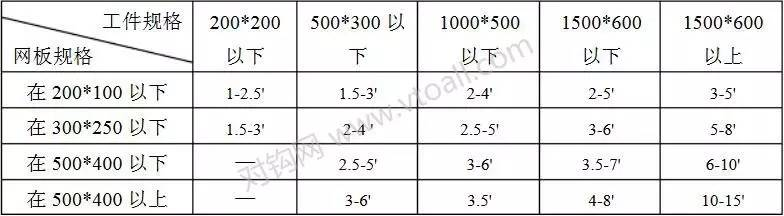（17）装配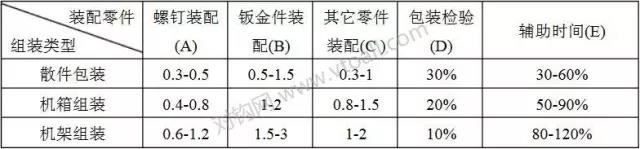• N1=螺钉数量

• N2=钣金件数量

• N3=其它零件数量

## 三、相关规定

1、在对照图纸定额加工工时时，可根据加工的难度和复杂性对其单件工时作以适当的调整。

2、须将产品的总工时成本控制在销售价的3-7%在内。

3、关于工时统计：

（1）一种料品一次性转序低于5件的不给予准备工时。

（2）连续s加工转序（即转序的间隔时间在一周以内）的产品一个工作单只给一次装备工时。